## Solving Rational Equations

An equation involving rational expressions is called a rational equation. To solve such an equation:

1. Completely factor all denominators.

2. Multiply both sides of the equation by the least common denominator (LCD).

3. Solve the resulting equation.

4. Eliminate any solutions that would make the LCD become zero. These solutions are called extraneous solutions.

5. Check the remaining solutions.

##### Example 1

Solve.

Factor all denominators.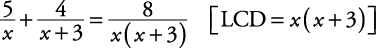Multiply both sides by LCD.Solve equation.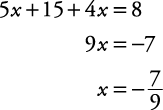Since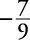is not an extraneous solution because it does not make the LCD zero, all that is needed is to verify thatis a solution to the original equation.

The check is left to you.

##### Example 2

Solve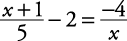.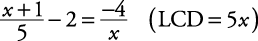Multiply both sides by LCD.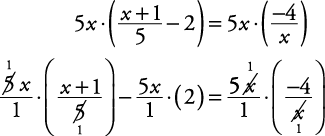Solve equation.

x 2 + x – 10 x = –20

Get all terms on one side and solve by factoring.Since neither answer is an extraneous solution, each must be checked with the original equation. The check of these solutions is left to you.